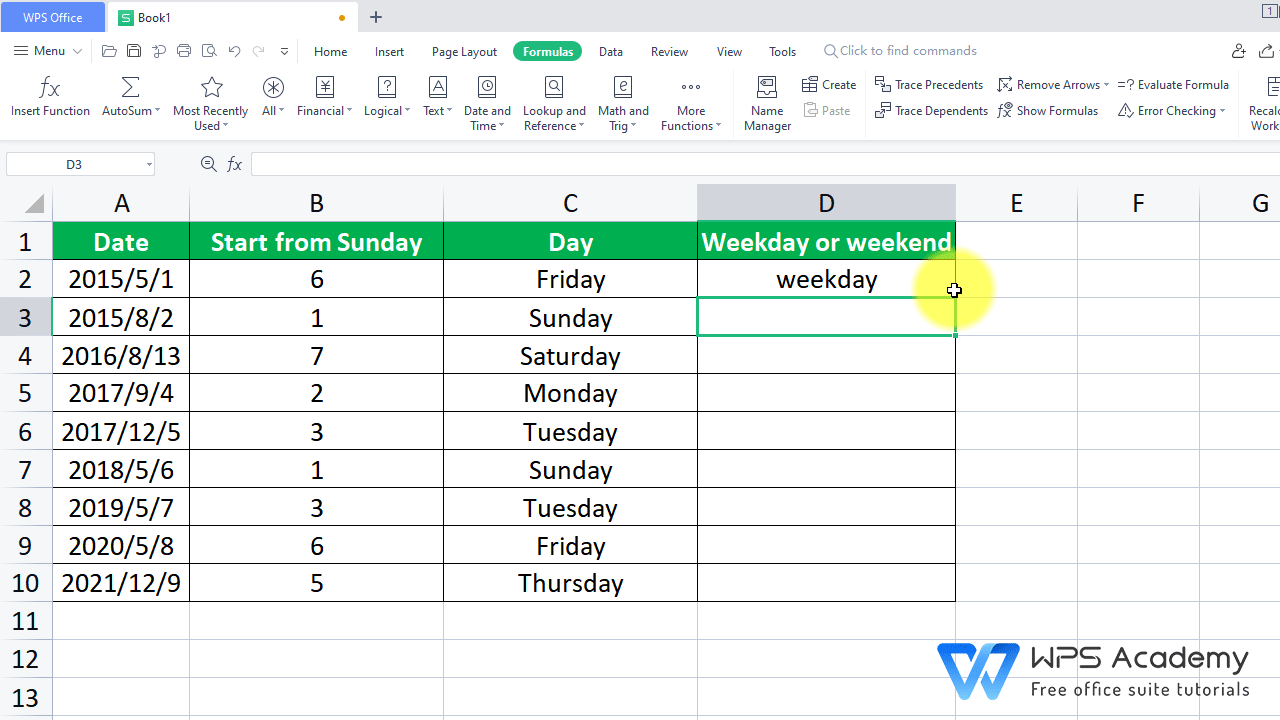The WEEKDAY Function in EXCEL

January 14, 2022
245 Views

Graphic skills

· Description:

Weekday Function can return a number from 1 to 7 identifying the day of the week of a date. In todays tutorial, we will also learn how to combine WEEKDAY with nested IF&OR function.

· Syntax:

(Serial_number, Return_type)

· Arguments:

Serial_number: The number that represents a date.

Return_type: You can enter a number here:

1: Returns a number from 1 to 7, meaning start from Sunday and end at Saturday. Its the default if omitted.

2: Returns a number from 1 to 7, meaning start from Monday and end at Sunday.

3: Returns a number from 0 to 6, meaning start from Monday and end at Sunday.

· Example:

Suppose we want to know the day of the week in column A, and identify whether it is weekday or weekend.

2. Select cell B2 where we want to insert the function. Then head to the Formulas tab > Insert Function > enter WEEKDAY in the edit box, or just type =WEEKDAY in the cell.

Here we need to input the WEEKDAY function.

Serial_number: The number that represents a date, A2 is the cell that contains the date, so let enter A2 here.

Return_type: 1: For Sunday through Saturday (1-7). Its the default if omitted.

We want to take Sunday as the first day of the week, so it is omitted. (1:Sunday, 2:Monday... 7:Saturday).

So, we enter A2 at Serial_number, and omit the second argument. Hove over cell B2 and drag it all the way down to complete B2 to B10.

We can see from the table that numerical values fill this column. It is still a little confused for us to get what the day is.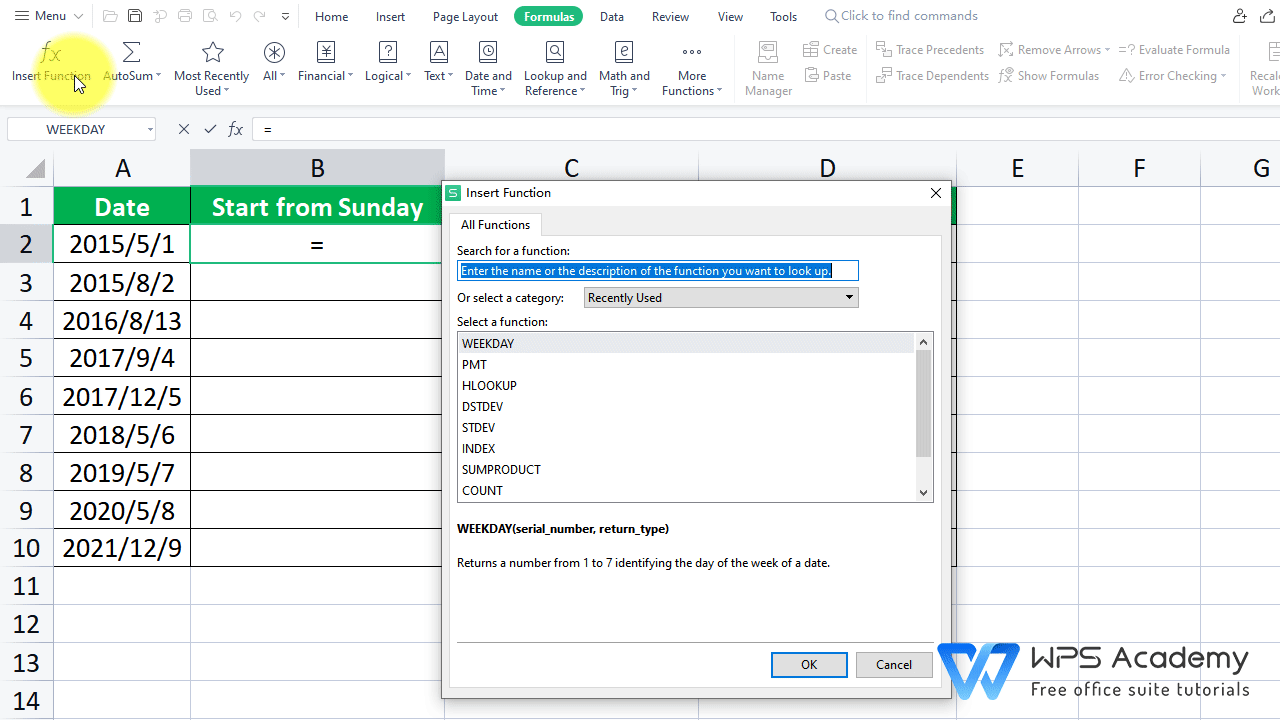3. Paste and copy the value of B2:B10 to C2:C10. Select C2:C10, then press Ctrl+1 to activate the Format Cells, where we can select Custom at Category.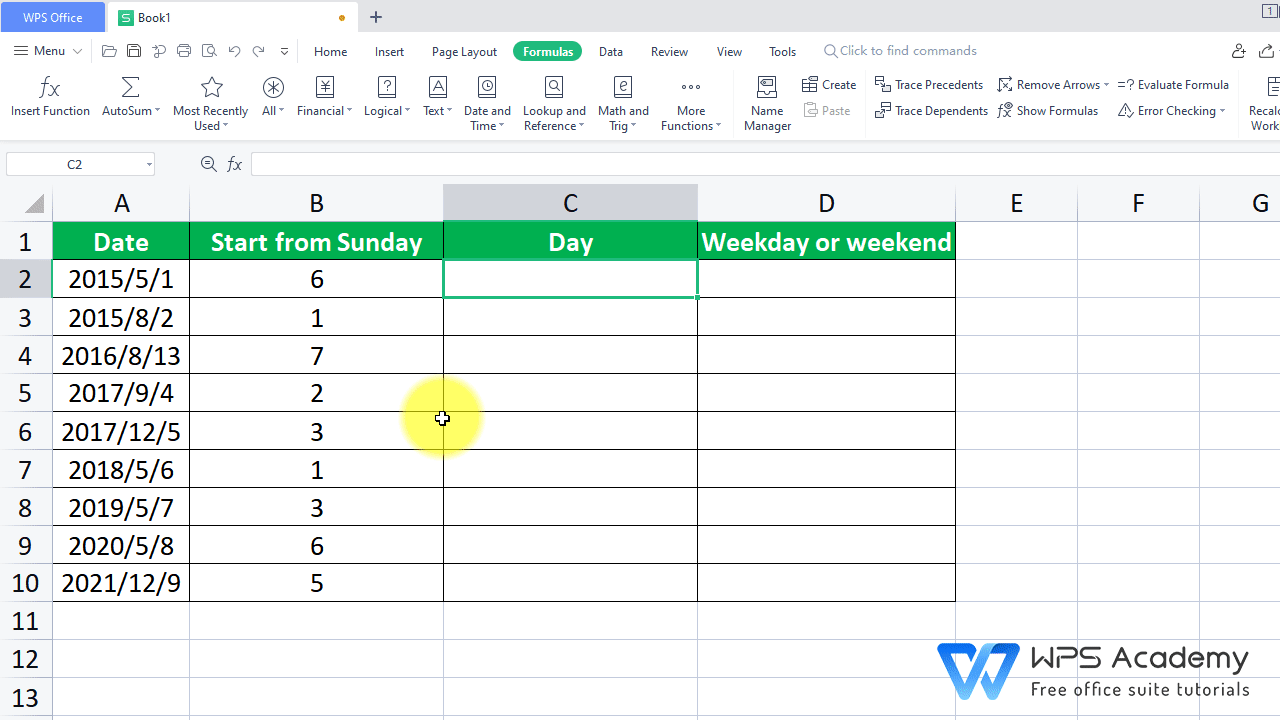4. Enter dddd at Type, the value in C2:C10 will generate the full name of the day, which will make the table more intuitive.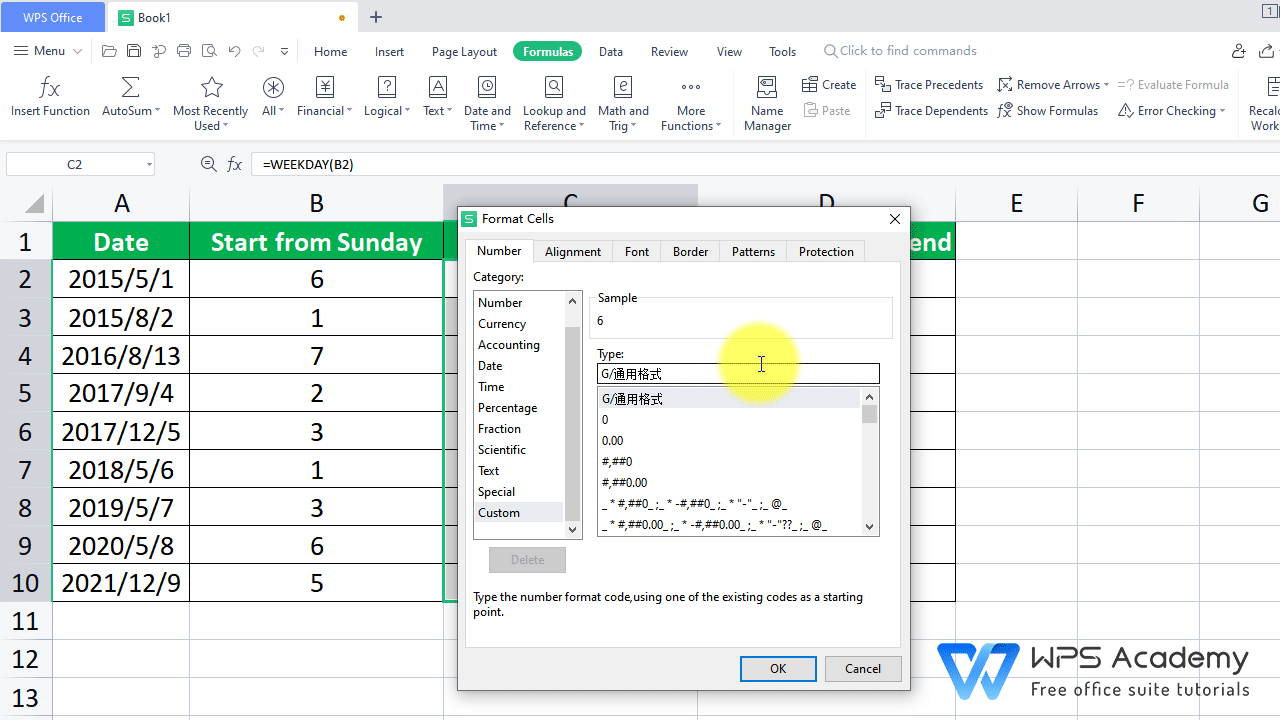5. Finally, how can we find out if the day is weekend or weekday? Nested IF&OR function can help us, which is quite simple. In this case, enter the following formula in cell D2:

=IF(OR(B2=1,B2=7),weekend,weekday).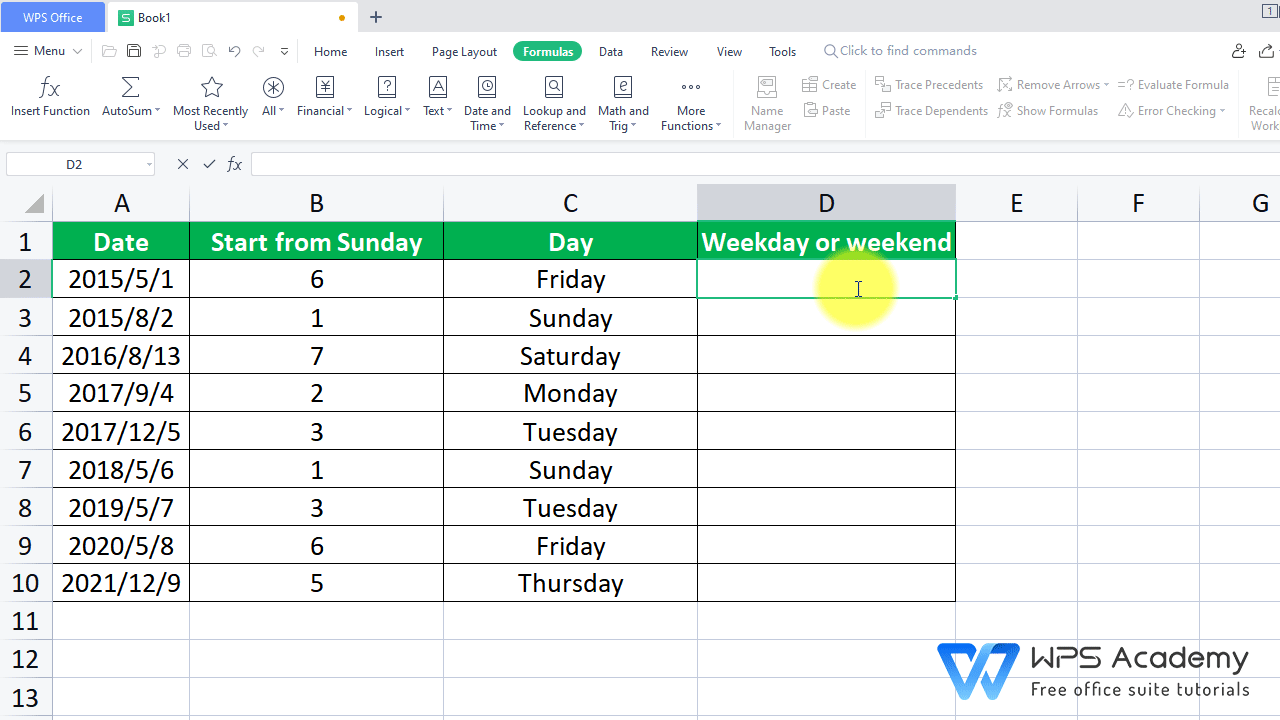Here is why we write this formula:

For the OR Function,OR function returns TRUE if any of the arguments is TRUE, and returns FALSE if all arguments are FALSE. We want to check if either B2=1 or B2=7 is satisfied, so those conditions are put in the OR Function.  1 stands for Sunday while 7 stands for Saturday.

IF function can check whether a condition is met, and returns one value if TRUE, in this case, if any condition of OR function is TRUE, the outside IF function will make the result  return weekend, otherwise, the IF function will return weekday.

The result returns Weekday, because 6 means the 6th day accounted from Sunday, that is, Friday.

Hove over cell D2 and drag it all the way down to complete D2 to D10. Up to this point, we have turned the table into what we want.